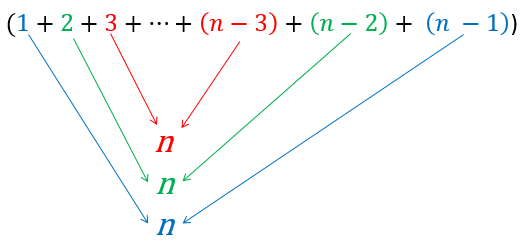# 8.13. Sorting Efficiency¶

To figure out how efficient the Selection Sort algorithm is, we will analyze the worst case. For units of work, we will consider comparisons and swaps - the two key operations in a sort. This video works through how much work there is for a Selection Sort on a list of 4 items:

Animation used by permission of Virginia Tech

If we did the same work for a selection sort on a list of 100 things ($$n = 100$$), it would look like:

Step

Comparisons

Swaps

1

99

1

2

98

1

3

97

1

97

3

1

98

2

1

99

1

1

The total swaps would be 99 steps times 1 swap each = 99. Expressed in terms of $$n$$ we could say it is $$n - 1$$ swaps.

The total comparisons would be 99 + 98 + 97 + … + 3 + 2 + 1 or $$(n - 1) + (n - 2) + (n - 3) + ... + 3 + 2 + 1$$. So what does that equal? There is a trick to find out - pair up the first item and the last, then the second item and next to last and so on. Each pair of items sums to $$n$$:Since there were $$n - 1$$ numbers to start with, there will be $$\frac{n - 1}{2}$$ pairs, each of which adds to $$n$$. Multiplying the number of pairs by the sum of each pair gives us the total: $$\frac{n - 1}{2} \cdot n = \frac{n^2 - n}{2} = \frac{n^2}{2} - \frac{n}{2}$$.

Overall, our work will be given by:

$$\text{Total work} = \text{Comparisons} + \text{Swaps}$$

Or:

$$\textrm{Total work} = (\frac{n^2}{2} - \frac{n}{2}) + (n-1) = \frac{n^2}{2} + \frac{n}{2} - 1$$

Since for Big-O purposes, all we care about is the class of the dominant term, in this case $$\frac{n^2}{2}$$, we will say that Selection Sort is $$O(n^2)$$ (Quadratic). A similar process can be used to show that so is Insertion Sort.

Important

Selection and Insertion Sort are Quadratic - $$O(n^2)$$.